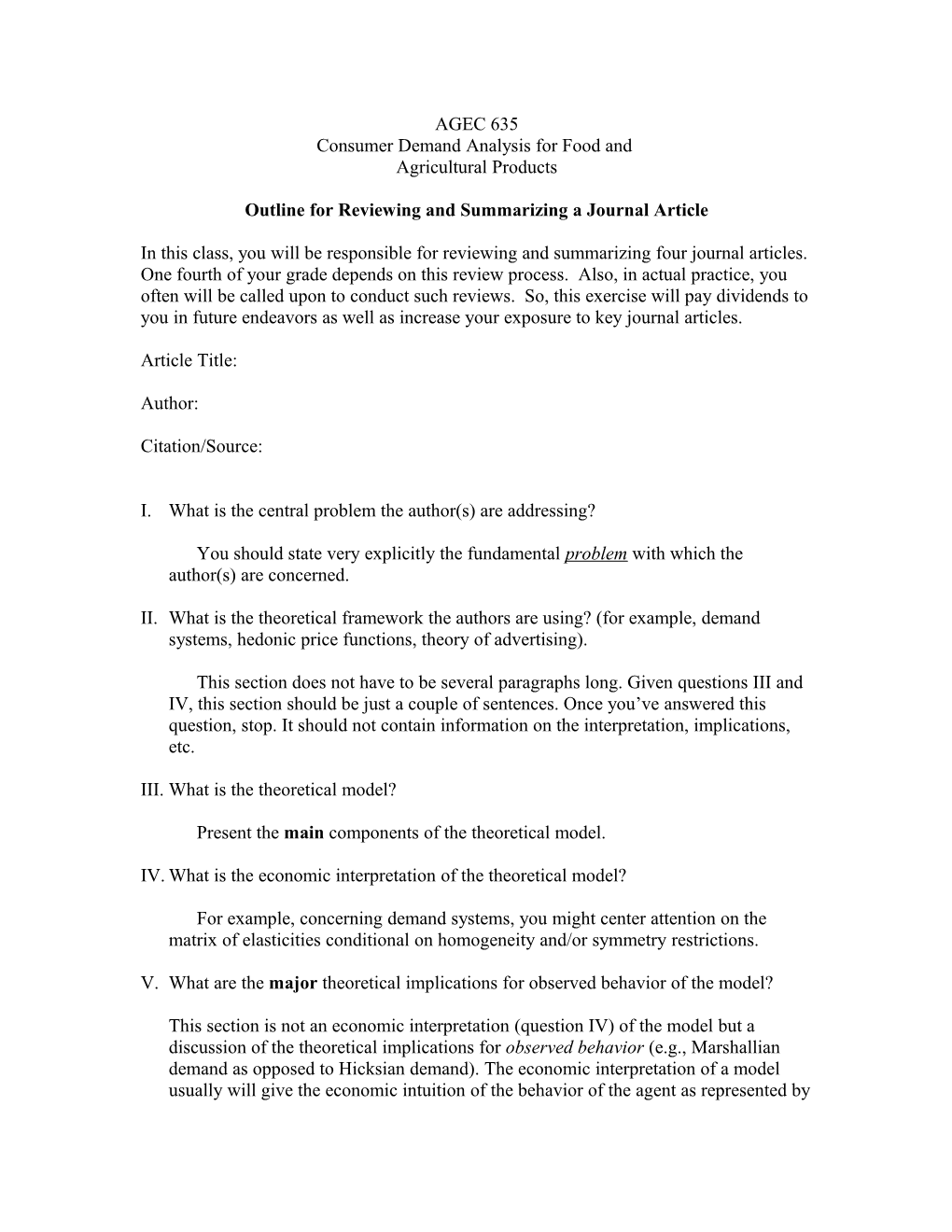# Outline for Reviewing and Summarizing a Journal ArticleAGEC 635

Consumer Demand Analysis for Food and

Agricultural Products

Outline for Reviewing and Summarizing a Journal Article

In this class, you will be responsible for reviewing and summarizing four journal articles. One fourth of your grade depends on this review process. Also, in actual practice, you often will be called upon to conduct such reviews. So, this exercise will pay dividends to you in future endeavors as well as increase your exposure to key journal articles.

Article Title:

Author:

Citation/Source:

1. What is the central problem the author(s) are addressing?

You should state very explicitly the fundamental problem with which the author(s) are concerned.

1. What is the theoretical framework the authors are using? (for example, demand systems, hedonic price functions, theory of advertising).

This section does not have to be several paragraphs long. Given questions III and IV, this section should be just a couple of sentences. Once you’ve answered this question, stop. It should not contain information on the interpretation, implications, etc.

1. What is the theoretical model?

Present the main components of the theoretical model.

1. What is the economic interpretation of the theoretical model?

For example, concerning demand systems, you might center attention on the matrix of elasticities conditional on homogeneity and/or symmetry restrictions.

1. What are the major theoretical implications for observed behavior of the model?

This section is not an economic interpretation (question IV) of the model but a discussion of the theoretical implications for observed behavior (e.g., Marshallian demand as opposed to Hicksian demand). The economic interpretation of a model usually will give the economic intuition of the behavior of the agent as represented by mathematical expressions. Usually these expressions will not be observed. For example, we do not observe in the market place first-order conditions, marginal utility, Lagrange multipliers, etc. All we observe are quantities consumed, prices paid, and resource constraints like income or time. However, underlying these observed variables we believe there is an economic story; for example, people will consume up to the point where marginal utility equals the shadow price of income times the price of the commodity. Provide an economic interpretation of the model. The implications refer to observed behavior and specifically what does the theoretical model imply about observed behavior in terms of comparative statics. For example, we know from the utility maximization model that a Marshallian demand function is homogeneous of degree zero in income and prices. That is an implication of the theory for observed behavior, since the homogeneity condition implies restrictions on the elasticities.

1. What is the empirical model?

By empirical model, describe the functional form, the dependent variable(s), the explanatory variables, etc.

1. What restrictions does the theory imply on the empirical model?

Think generally. Do you expect parameters to be positive or negative, i.e. what are the expected signs stemming from the theory?

1. What are the data and data sources?

This section should only be a few sentences.

1. What major contributions to the literature does this make?

How is it different from previous articles and how does it advance the literature?

This section should not be a summary.

1. What are the major limitations of the model that you can think of?